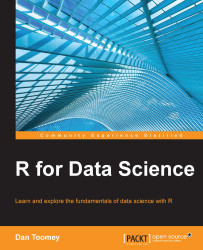•#### R for Data Science#### Overview of this book

R for Data ScienceCreditswww.PacktPub.comPrefaceFree Chapter
Data Mining PatternsData Mining SequencesText MiningData Analysis – Regression AnalysisData Analysis – CorrelationData Analysis – ClusteringData Visualization – R GraphicsData Visualization – PlottingData Visualization – 3DMachine Learning in ActionPredicting Events with Machine LearningSupervised and Unsupervised LearningIndex## Questions

Factual

• How can you decide whether to use Pearson, Kendall, or Spearman as a method for correlation?

• When would you want to see a small degree of freedom in the correlation results?

• Most of the examples used common default parameters. Explore the same examples with nondefault parameters.

When, how, and why?

• Why do the polychoric functions take so long to process?

• Why are the values chosen in the polychoric responses correlated?

• Explain the threshold values that were calculated.

Challenges

• Is there an easier way to develop the 2 x 2 matrix needed as input to the tetrachoric function?

• How could you account for trends in investment vehicles when analyzing stock market returns versus fixed instruments?